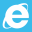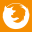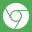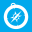VintaSoft Imaging .NET SDK v9.1 for Web
Vintasoft.Imaging.ImageProcessing Namespace / WebClearCommandJS type / set_Region Method
Coordinate of the left top corner of the rectangular region of interest. Valid value is 0 and any positive value. Default value is 0.
Coordinate of the left top corner of the rectangular region of interest. Valid value is 0 and any positive value. Default value is 0.
Width of the rectangular region of interest. Valid value is 0 and any positive value. Default value is 0.
Height of the rectangular region of interest. Valid value is 0 and any positive value. Default value is 0.
In This Topic
set_Region Method
In This Topic
Sets an image region, which should be processed by the image processing command.
Syntax
```var instance = new Vintasoft.Imaging.ImageProcessing.WebClearCommandJS();
var value; // Type: any

// Parameters
var x; // Type:  number
var y; // Type:  number
var w; // Type:  number
var h; // Type:  number

value = instance.set_Region(x, y, w, h);```
```function set_Region(
x : number,
y : number,
w : number,
h : number
) : any;```

#### Parameters

x
Coordinate of the left top corner of the rectangular region of interest. Valid value is 0 and any positive value. Default value is 0.
y
Coordinate of the left top corner of the rectangular region of interest. Valid value is 0 and any positive value. Default value is 0.
w
Width of the rectangular region of interest. Valid value is 0 and any positive value. Default value is 0.
h
Height of the rectangular region of interest. Valid value is 0 and any positive value. Default value is 0.
Exceptions
ExceptionDescription
Thrown if arguments have wrong types.
Browser Compatibility95155